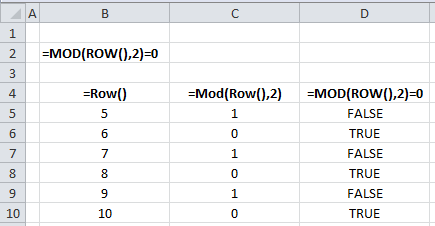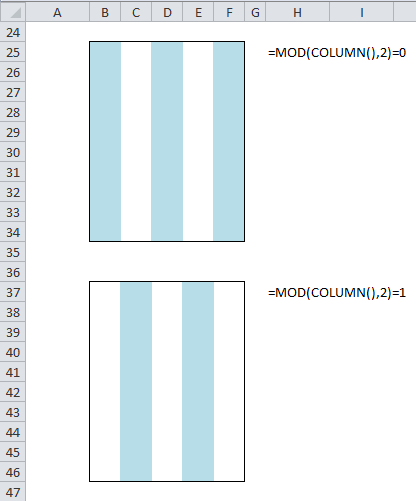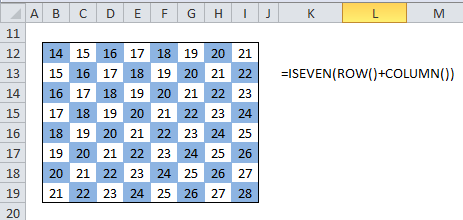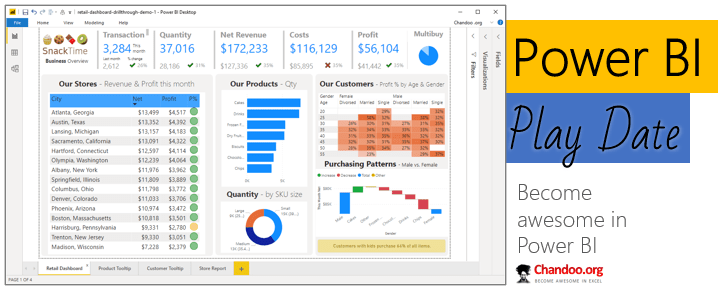# Formula Forensics No. 005 – Zebras and Checker-Boards

Share

This week in Formula Forensics we’ll look at, Zebra Stripes and Checker-board Conditional Formatting.

This idea is inspired by a number of posts over the past few years asking about zebra stripes but specifically BobR who in in June 2011, also asked about Checkerboards in the post: Want to be an excel conditional-formatting Rock Star, Comment No. 154.

I got the conditional format for alternating row and column colors,

Is there a conditional format to make it a checkerboard whereas the cell A2 will remove either the conditional for the row or column and then alternately to A4, B1, B3 etc?

Chandoo responded fairly quickly with this Conditional Formatting formula:

=IF(MOD(ROW(),2)=1,MOD((ROW()-1)*8+COLUMN(),2)=0,MOD((ROW()-1)*8+COLUMN(),2)=1)

Unbeknownst to Chandoo I posted this about a minute later:

=ISODD(ROW()+COLUMN())

Both formula correctly answer BobR’s question.

So today we’re going to pull apart Zebra Stripes and Checker Boards and see what makes them tick.

## ZEBRA STRIPES

Zebra Stripes as Conditional Formatting is simply applied using a simple formula within Conditional Formatting.

=MOD(ROW(),2)=0

Conditional Formatting requires a formula that returns a boolean “True” to apply a format or a Boolean “False” to not Apply a format.

So the formula is better read as: If MOD(ROW(),2)=0

And  If MOD(ROW(),2)=0, the formula will evaluate as True

This is best evaluated as 3 columns on a worksheet.In cells

B5:B10 The formula =Row() returns the Row Number

C5:C10 The formula =Mod(Row() ,2) returns the Mod of Row Number, divided by 2

The Mod function returns the remainder of the division of the Row Number divided by 2,

So in Row 5, Mod(Row(),2) = Mod(5, 2) = 5/2 = 2 Remainder 1 = 1

and in Row 6, Mod(Row(),2) = Mod(6, 2) = 6/2 = 3 Remainder 0 = 0

D5:D10 The formula =Mod(Row() ,2)=0 checks the remainder against the value 0

This is what evaluates to either True or False depending on the Row number.

Where the Values are True the Format will be applied (Even Rows)The Conditional Formatting can be applied to Odd Rows If the Formula is slightly altered

=Mod(Row() ,2)=1Similarly the formatting can be applied to Columns using

=MOD(COLUMN(),2)=0/1## CHECKER BOARDS

RobR received two responses to his Checker-Board Conditional Formatting request.

=IF(MOD(ROW(),2)=1,MOD((ROW()-1)*8+COLUMN(),2)=0,MOD((ROW()-1)*8+COLUMN(),2)=1)

and

=ISODD(ROW()+COLUMN())

Lest see what’s inside these two formula.

## =IF(MOD(ROW(),2)=1,MOD((ROW()-1)*8+COLUMN(),2) =0, MOD( (ROW() -1)*8+COLUMN(),2)=1)

This is a simple If Formula with 3 components

=IF(MOD(ROW(),2)=1,MOD((ROW()-1)*8+COLUMN(),2)=0,MOD((ROW()-1)*8+COLUMN(),2)=1)

If Condition        MOD(ROW(),2)=1

Value if True:     MOD((ROW()-1)*8+COLUMN(),2)=0

Value if False:    MOD((ROW()-1)*8+COLUMN(),2)=1

The If Condition is already known to us, as it’s the same formula used in the Zebra Stripes above.

It evaluates to True when it is on an Odd Row.

So when it is an Odd numbered Row Excel will look at MOD((ROW()-1)*8+COLUMN(),2)=0

And when it is an Even numbered Row Excel will look at MOD((ROW()-1)*8+COLUMN(),2)=1

We can notice that these are the same formulas which have a different ending of =0 and =1

MOD((ROW()-1)*8+COLUMN(),2)=0

This section Takes each Row subtracts 1 and then multiplies this number by 8. This can be expressed as simply as saying multiply the Row * 8.

This will always return an Even Number and could have been simplified to Row()*2

MOD((ROW()-1)*8+COLUMN(),2)=0

The next bit adds the column number to the previous Even Number.

So now this part will be Odd when the column is Odd and Even when the column is Even.

MOD((ROW()-1)*8+COLUMN(),2)=0

The remainder of the formula is the same as the Zebra Stripes formula.

An Odd Number (Odd Columns) in the section above will return a 1 as the result of =Mod(Odd,2)

An Even Number (Even Columns) in the section above will return a 0 as the result of =Mod(Odd,2)

When evaluated against 0 will return True for Even Columns and False for Odd Columns.

Now the exact same happens in the False section of the If formula except that it is evaluated against 1.

## =ISODD(ROW()+COLUMN())

I tackled this problem from a different direction to Chandoo.

Knowing that Even + Even = Even and Even + Odd = Odd and that the row and Column Numbers increase in each direction by 1 each Row/Column, it was simply a matter of adding the Row and Column numbers together and checking if it was Odd or Even

The Excel function IsOdd() and IsEven() both return a Boolean “True” if the contents are Odd or “Even” respectively. This negates an external truth check as described above.

This is easily shown by adding a formula to the Checker area

=Row()+Column()Excel 2003: The above formula won’t work in Excel 2003.

If the alternate shading is required a switch to

=ISEVEN(ROW()+COLUMN())

Does the trick.Excel 2003: The above formula won’t work in Excel 2003.

http://chandoo.org/wp/2009/03/13/excel-conditional-formatting-basics/

and

and

http://chandoo.org/wp/2008/10/14/more-than-3-conditional-formats-in-excel/

## OTHER POSTS IN THIS SERIES

Formula Forensics 001 – Tarun’s Problem

Formula Forensics 002 – Joyce’s Question

Formula Forensics 003 – Lukes Reward

Formula Forensics 004 – Freds Problem

## We Need Your Help !

If you have a neat formula that you would like to share and explain, try putting pen to paper and draft up a Post as Luke did in Formula Forensics 003. or this post.

If you have a formula that you don’t understand and would like explained but don’t want to write a post also send it in to Chandoo or Hui.

### Get FREE Excel + Power BI Tips

Simple, fun and useful emails, once per week.

Learn & be awesome.

### Welcome to Chandoo.org

Thank you so much for visiting. My aim is to make you awesome in Excel & Power BI. I do this by sharing videos, tips, examples and downloads on this website. There are more than 1,000 pages with all things Excel, Power BI, Dashboards & VBA here. Go ahead and spend few minutes to be AWESOME.

Read my storyFREE Excel tips book

Chandoo is an awesome teacher
5/5

– Jason

Still on fence about Power BI? In this getting started guide, learn what is Power BI, how to get it and how to create your first report from scratch.### Introducing Power BI Play Date – Online class to master Power BI (oh yeah, Power Query & Power Pivot too)

Hello friends,

Check out below slideshow to see some of the reports you can create in our course. It is #AWESOME.

## Related Tips

### 17 Responses to “Formula Forensics No. 005 – Zebras and Checker-Boards”

1.Nate says:

The links are missing the WP/ in them...

2.Hui... says:

Thanx Nate, All fixed.
Strangely they all worked this afternoon as I actually checked them all myself, before it was published ?

3.Fred says:

Thanks, Hui! Love it! what a neat trick! Awesome!

4.Jeff Weir says:

=Row()+Column() is sheer genius!

5.Nithya Srivatsan says:

This is really awesome..I love this website..Not sure if I can talk to one person to resolve the issues that I have with excel and formulas.

6.Hui... says:

@All
.
@Nithya
Can you post your question on the Chandoo.org Forums ?
http://chandoo.org/forums/?new=1

7.Delain says:

In my Excel 2003, using =ISODD(ROW()+COLUMN()) as formula for Conditional Formatting,
I receive an error msg of "You may not use references to other worksheets or workbooks
for Conditonal Formatting criteria.
I can use the formula as a cell formula just fine; shows True/False as expected.
Thoughts?

8.daffy333 says:

This is great! BUT zebra rows give me headaches...

However, double-wide rows are a little more pleasant to the eyeballs. =ISEVEN((ROW()+X)/2) will give you double-wide zebras, and you place a number in X to influence the sequence of stripes. Replace /2 with /3 if you want them 3 wide, etc etc.

Happy Zebra'ing!

9.moatasem says:

@Delain
In order to use =ISODD(ROW()+COLUMN()) in Excel 97-2003,
You have to activate the Analysis ToolPak first from Tools » Add-ins to be able to use ISODD and ISEVEN Functions.

10.Delain says:

Thanks for the suggestion, moatasem.
However, I've had the ToolPak activated for a long time and as you can see from my original post, I can use it as a formula in a worksheet cell, but will not function without an error msg in Conditional Formatting. So, my issue persists.
I would appreciate other suggestions to solve.

•Rej says:

@Delain, it's posted above
Excel 2003: The above formula won’t work in Excel 2003.

11.Hui... says:

@Delain
Your correct Excel 2003 doesn't like the use of =ISODD(ROW()+COLUMN()) as a Conditional Format formula. I can't answer why.
.
However as always there is an alternative, try:

=Mod(Row()+Column(),2)=0
or
=Mod(Row()+Column(),2)=1

12.Rana says:

Hi Guys,

Sorry to start a new thread. Need help with Conditional formatting.
Column D has a drop down list, column H & J have start and end date. Need formula to conditionaly format cells and change colour for row m2:x2, such that if D2= "Completed", then considering the date range in H2 & J2 it should colour format specific months for row m2:x2.

I am currenly trying to use - =IF(D\$2="Completed",AND(M\$1>=\$H2,M\$1<=\$J2))

M1 = 1/Sep/2011
H1 = 1/sep/2011
J2 = 10/Oct/2011

13.Hui... says:

@Rana
Can you post your file or part of it?

14.Rana says:

Sorry - am new but how do I post it? Can I send it to chandoo.g@gmail.com?

15. [...] Zebra Stripes and Checker-Board – Conditional Formatting – Chandoo [...]

16.Nate says:

In Chandoo's formula above, you can eliminate the entire (ROW()-1)*8 section and you will still end up with zeba stripes. If we were to consider Rows 3 and 4, we would evaluate the formula as follows:

Row 3: IF(MOD(ROW(),2)=1 Returns TRUE
(ROW()-1)*8+COLUMN() = 0 then becomes
(3-1)*8+COLUMN() = 0
16+COLUMN() = 0
As the (ROW()-1)*8 will always return an even number, the formula depends entirely on the result of COLUMN() to determine the outcome.

Row 4: IF(MOD(ROW(),2)=1 Returns FALSE
(ROW()-1)*8+COLUMN() = 1 then becomes
(4-1)*8+COLUMN() = 0
24+COLUMN() = 0
Again, the (ROW()-1)*8 has returned an even number and always will. The result of the formula is once more entirely dependent on the COLUMN().

The same holds true for any row.

The logic in the simplified formula has become:
=IF(ISODD(ROW()),ISEVEN(COLUMN()),ISODD(COLUMN())
This will return a TRUE as long as one of the two values is odd.

### Get FREE Excel & Power-BI Newsletter

One email per week with Excel and Power BI goodness. Join 100,000+ others and get it free.